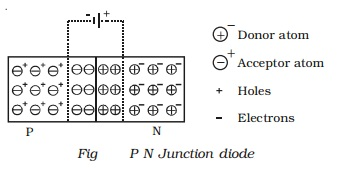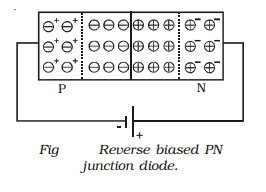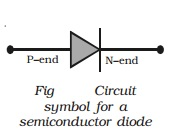Home | | Physics | PN Junction diode: Forward and Reverse bias characteristics

# PN Junction diode: Forward and Reverse bias characteristicsIf one side of a single crystal of pure semiconductor (Ge or Si) is doped with acceptor impurity atoms and the other side is doped with donor impurity atoms, a PN junction is formed as shown in Fig. P region has a high concentration of holes and N region contains a large number of electrons.

PN Junction diode

If one side of a single crystal of pure semiconductor (Ge or Si) is doped with acceptor impurity atoms and the other side is doped with donor impurity atoms, a PN junction is formed as shown in Fig. P region has a high concentration of holes and N region contains a large number of electrons.As soon as the junction is formed, free electrons and holes cross through the junction by the process of diffusion. During this process, the electrons crossing the junction from N-region into the P region, recombine with holes in the P-region very close to the junction. Similarly holes crossing the junction from the P-region into the N-region, recombine with electrons in the N-region very close to the junction. Thus a region is formed, which does not have any mobile charges very close to the junction. This region is called depletion region. In this region, on the left side of the junction, the acceptor atoms become negative ions and on the right side of the junction, the donor atoms become positive ions (Fig).

An electric field is set up, between the donor and acceptor ions in the depletion region. The potential at the N-side is higher than the potential at P-side. Therefore electrons in the N-side are prevented to go to the lower potential of P-side. Similarly, holes in the P-side find themselves at a lower potential and are prevented to cross to the N-side. Thus, there is a barrier at the junction which opposes the movement of the majority charge carriers. The difference of potential from one side of the barrier to the other side is called potential barrier. The potential barrier is approximately 0.7V for a silicon PN junction and 0.3V for a germanium PN junction. The distance from one side of the barrier to the other side is called the width of the barrier, which depends upon the nature of the material.

Forward biased PN junction diode

When the positive terminal of the battery is connected to P-side and negative terminal to the N-side, so that the potential difference acts in opposite direction to the barrier potential, then the PN junction diode is said to be forward biased.

When the PN junction is forward biased (Fig), the  applied positive potential repels the holes in the P-region, and the applied negative potential repels the electrons in the N-region, so the charges move towards the junction. If the applied potential difference is more than the potential barrier, some holes and free electrons enter the depletion region.Hence, the potential barrier as well as the width of the depletion region are reduced. The positive donor ions and negative acceptor ions within the depletion region regain electrons and holes respectively. As a result of this, the depletion region disappears and the potential barrier also disappears. Hence, under the action of the forward potential difference, the majority charge carriers flow across the junction in opposite direction and constitute current flow in the forward direction.

Reverse biased PN junction diode

When the positive terminal of the battery is connected to the N-side and negative terminal to the P-side, so that the applied potential difference is in the same direction as that of barrier potential, the junction is said to be reverse biased.

When the PN junction is reverse biased (Fig), electrons in the N region and holes in the P-region are attracted away from the junction.

Because of this, the number of negative ions in the P-region and positive ions in the N-region increases. Hence the depletion region becomes wider and the potential barrier is increased.Since the depletion region does not contain majority charge carriers, it acts like an insulator. Therefore, no current should flow in the external circuit. But, in practice, a very small current of the order of few microamperes flows in the reverse direction. This is due to the minority carriers flowing in the opposite direction. This reverse current is small, because the number of minority carriers in both regions is very small. Since the major source of minority carriers is, thermally broken covalent bonds, the reverse current mainly depends on the junction temperature.

Symbol for a semiconductor diode

The diode symbol is shown in Fig. The P-type and N-type regions are referred to as P-end and N-end respectively. The arrow on the diode points the direction of conventional current.Forward bias characteristics

The circuit for the study of forward bias characteristics of PN junction diode is shown in Fig a. The voltage between P-end and N-end is increased from zero in suitable equal steps and the corresponding currents are noted down. Fig b shows the forward bias characteristic curve of the diode. Voltage is the independent variable. Therefore, it is plotted along X-axis. Since, current is the dependent variable, it is plotted against Y-axis. From thecharacteristic curve, the following conclusions can be made. (i) The forward characteristic is not a straight line. Hence the ratio V/I is not a constant (i.e) the diode does not obey Ohm's law. This implies that the semiconductor diode is a non-linear conductor of electricity. (ii) It can be seen from the characteristic curve that initially, the current is very small. This is because, the diode will start conducting, only when the external voltage overcomes the barrier potential (0.7V for silicon diode). As the voltage is increased to 0.7 V, large number of free electrons and holes start crossing the junction. Above 0.7V, the current increases rapidly. The voltage at which the current starts to increase rapidly is known as cut-in voltage or knee voltage of the diode.

Reverse bias characteristics

The circuit for the study of reverse bias characteristics of PN junction diode is shown in Fig a. The voltage is increased from zero in suitable steps. For each voltage, the corresponding current readings are noted down. Fig b shows the reverse bias characteristic curve of the diode. From the characteristic curve, it can be concluded that, as voltage is increased from zero, reverse current (in the order of microamperes) increases and reaches the maximum value at a small value of the reverse voltage. When the voltage is further increased, the current is almost independent of the reverse voltage upto a certain critical value. This reverse current is known as the reverse saturation current or leakage current. This current is due to the minority charge carriers, which depends on junction temperature.Study Material, Lecturing Notes, Assignment, Reference, Wiki description explanation, brief detail
11th 12th std standard Class Physics sciense Higher secondary school College Notes : PN Junction diode: Forward and Reverse bias characteristics |